## Saturday, 30 May 2020

### The rise of the "method"

As I write, I have been engaged in a discussion on twitter around "methods" for decomposing a number into the product of its prime factors. The thread is interesting enough and can be found from this tweet.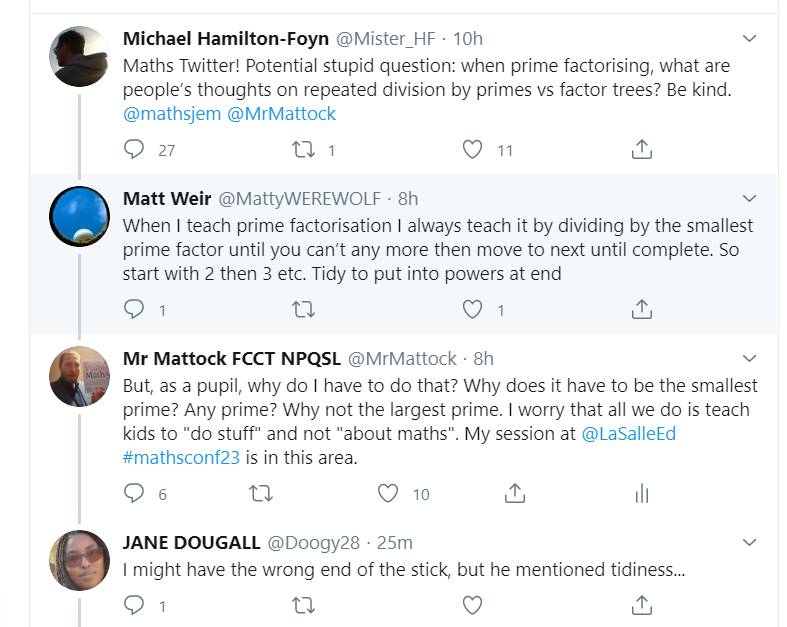Now I have no problem with discussions around methods. Each one is an interesting mathematical process, and there are often pros and cons between one "method" and another for doing whatever it is you want to do. I love @mathsjem's book that examines different methods for doing different things.

However, I feel there is a real danger arising in maths teaching whereby teachers think that in teaching a collection of methods, they are teaching mathematics. Even those that purport to teach "mutliple methods" are, I feel, missing the point. The point being that the method is not the mathematics. No matter how much conceptual understanding runs alongside it, the method itself cannot be the mathematics. And this is because the mathematics is that structure, that idea, from which the method arises.

Take, for example, the above mentioned "prime factorisation". There are two mathematical ideas at play in this - namely "prime" and "factorisation". What are these ideas? What is the structure that underpins them. Let us deal with the second one first, because that is required to understand the nature of "prime". What is factorisation? What do I understand by this idea?

Simply put, factorisation is the manipulation of an expression to write it as the product of two or more factors. Of course, this leads us further to ask what a factor is, and from there further down. There are lots of representations and manipulatives that can support in developing these ideas that are explored heavily in Visible Maths, so I am not going to re-hash them here. The key is, that this is the underlying structure, and it is true no matter when the word "factorisation" is used:

3 × 6 is a factorisation of 18.
2 × 3 × 3 is a factorisation of 18.
3x × 6 is a factorisation of 18x.
6(3x + 5) is a factorisation of 18x + 30.

It is this key understanding of what factorisation is that pupils need to understand, so that they can apply it no matter what the context. If I understand this, then any "method" I use for factorisation has to be taken in the context that this is what it is accomplishing - the decomposition into the product of two factors. Personally I would choose methods that appear the same/similar across as many contexts as possible, which is why I have moved away from things like "the factor tree" as it is typically only used to accomplish prime factor decomposition.

Once we have a clear idea of what it means to "factorise", we are in a position to understand primes as those numbers greater than 1 that cannot be factorised using numbers greater than 1 (or however you want to define primes that results in the same outcome). Again, there are lots of ways of exploring this idea with pupils - the Sieve of Eratosthenes being one of my favourites and the idea of factor towers being another:With a thorough understanding of these two concepts, then the idea of "prime factorisation" becomes self-evident (or if it doesn't, easily linked to the two concepts already covered). A prime factorisation must be a factorisation that only involves prime numbers. Nothing else makes sense. so 3 × 6 is not a prime factorisation of 18 as 6 is not prime, but 2 × 3 × 3 is. At this point, the "method" for actually carrying out the decomposition is almost immaterial - as long as it is clear how it does the job it is is supposed to be doing. On this I stand firmly with @jemmaths, that the method should not be designed to insulate from the mathematics, but rather to highlight it. This, again is where I feel factor trees fall down - they not only obscure the links between prime factorisation and other factorisations, but they also obscure that it is factorisation that is happening at all!

For another example, take the simple method of "column addition". Again two ideas are a play here, namely the idea of addition, and the idea of place value. If, in isolation from each other, I am helped to understand that a way of making sense of addition is the collection of like objects, and that place value uses numerals in different columns to represent the size of different parts of a number (as well as the relationships between these columns), then addition in columns for larger numbers follows naturally. Not only does it follow naturally, but it is adaptable. I can see why this calculation can be done in columns in any order: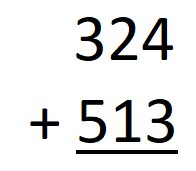whereas it might be beneficial to do this one from right to left: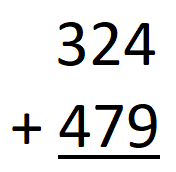but that if I do complete it right to left, I can see what has gone on: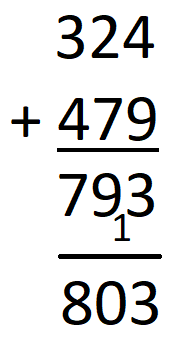and also why I might not use column addition at all: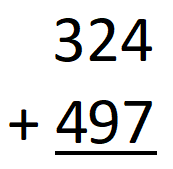If you are teaching maths, I implore you to actually teach maths. Teach kids about what is happening when you add, or factorise. Teach them what it means to be prime, or how place value works. Then teach them one or more methods that arise from these understandings.